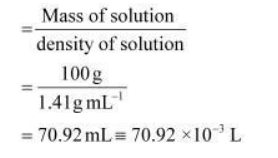# Calculate the concentration of nitric acid in moles

Question.

Calculate the concentration of nitric acid in moles per litre in a sample which has a density, $1.41 \mathrm{~g} \mathrm{~mL}^{-1}$ and the mass per cent of nitric acid in it being $69 \%$.

Solution:

Mass percent of nitric acid in the sample = 69 % [Given]

Thus, 100 g of nitric acid contains 69 g of nitric acid by mass.

Molar mass of nitric acid (HNO3)

$=\{1+14+3(16)\} \mathrm{g} \mathrm{mol}^{-1}$

= 1 + 14 + 48

$=63 \mathrm{~g} \mathrm{~mol}^{-1}$

Number of moles in $69 \mathrm{~g}$ of $\mathrm{HNO}_{3}$

$=\frac{69 \mathrm{~g}}{63 \mathrm{~g} \mathrm{~mol}^{-1}}$ $=1.095 \mathrm{~mol}$

Volume of 100g of nitric acid solutionConcentration of nitric acid

$=\frac{1.095 \mathrm{~mole}}{70.92 \times 10^{-3} \mathrm{~L}}$ $=15.44 \mathrm{~mol} / \mathrm{L}$

com5Concentration of nitric acid = 15.44 mol/L